# Applications of Op Amp

A linear amplifier like an op amp has many different applications. It has a high open loop gain, high input impedance and low output impedance. It has high common mode rejection ratio. Due to these favourable characteristics, it is used for different application. In this article, we are discussing some of the most prominent uses of an Op amp. This is not an exhaustive list but covers the important applications of op amp within the scope of our discussion.

## Op Amp applications as Inverting Amplifiers

Op-Amp can be used as an inverting amplifier.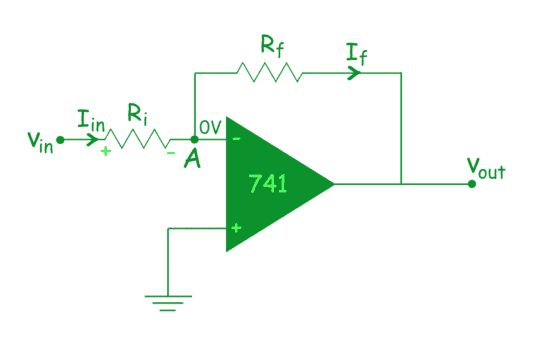• The inverting circuits, implemented with an Op-Amp, are more constant, distortion is comparatively lower, provide a better transitory response.
• When Op-Amp is applied in a closed loop, there is a linear relationship between input and output.
• The inverting amplifier can be applied for unity gain if Rf = Ri (where, Rf is the feedback resistor and Ri is input resistor)

## Op Amp Applications as Non Inverting Amplifiers

The input signal when applied to the non-inverting input (+), the output is applied back to the input via the feedback circuit created by Rf and Ri (where, Rf is the feedback resistor and Ri is input resistance).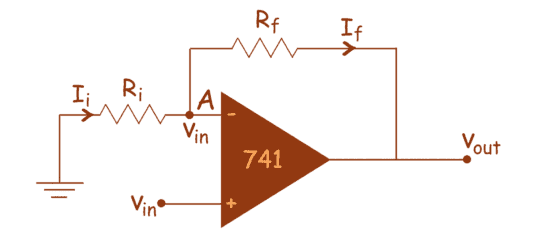• Voltage gain without any kind of phase inversion. In transistor equivalent, there are minimum 2 transistor stages needed to do this.
• High input impedance compared to Inverting input.
• The total remoteness of the signal supply from the output.

## Op Amp application as a Phase Shifter

Op-Amp is used for direct coupling procedure and so DC voltage level at the emitter terminal increases from phase to phase. This rapidly increasing DC level is likely to shift the operating point of the upcoming stages. Thus to move down the increasing voltage swing, this phase shifter is applied.The phase shifter performs by adding a DC voltage level to the output of fall stage to pass the output to a ground level.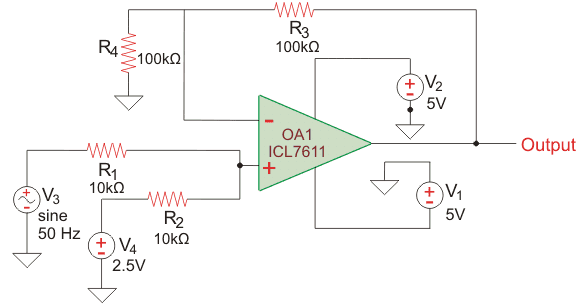## Op Amp as Scale Changer

Op-Amp functions as a scale changer through small signals with constant-gain in both inverting and non-inverting amplifiers.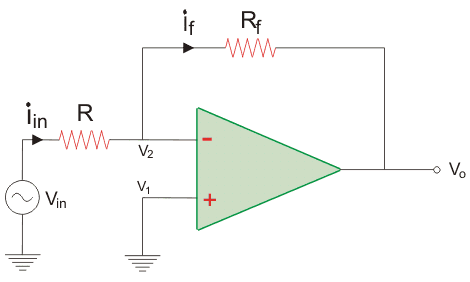Non-inverting terminal is grounded whereas R1 links the input signal v1 to the inverting input. A feedback resistor Rf is then connected from output to the inverting input. The closed loop gain of the inverting amplifier works based on the ratio of the two external resistors R1 and Rf and Op-Amp acts as a negative scaler when it multiplies the input by a negative constant factor.
While in need for an output that is equal to input for getting multiplied by a positive constant the positive scaler circuit is used by applying negative feedback.

## Op Amp Applications as Adder or Summing Amplifier

Op-amp can be used to sum the input voltage of two or more sources into a single output voltage. Below is a circuit diagram depicting the application of an op-amp as an adder or summing amplifier. The input voltages are applied to the inverting terminal of the op-amp. The inverting terminal is grounded. The output voltage is proportional to the sum of the input voltages.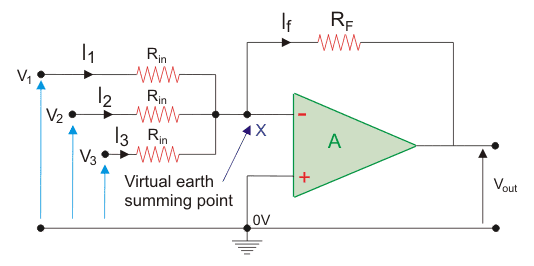## Op Amp Applications as a Differential Amplifier

Differential Amplifier is a useful blend of both the inverting amplifier and non-inverting amplifier. It is mostly used to amplify the diversity amid two input signals.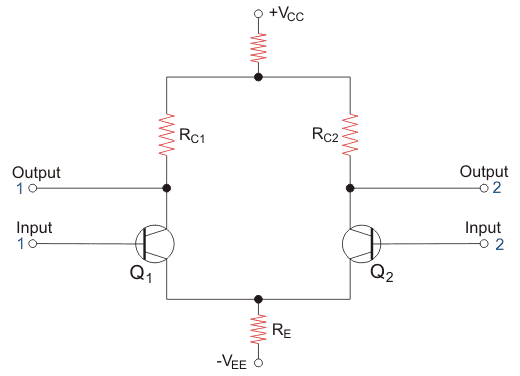Major applications of Differential Amplifiers are

• Signal Amplification
• Input stage emitter coupled logic
• Switch
• Controlling of Motors and Servo Motors

Example: it is useful while eliminating the noise in ambience as through differential amplifier, you can eliminate the connected protected cable or twisted pair cable mostly used to eradicate the transitory noise.

## Op Amp application as a Differentiator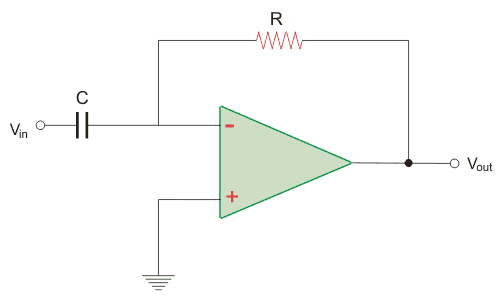Op-amp can be used as a differentiator where the output is the first derivative of the input signal. The following equation gives the relation between the input signal and the output signal.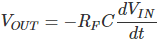As you can see the output voltage is a first derivative of the input voltage. We are not going into how the equation is derived but only learning about the use of an op amp as a differentiator.

## Op Amp Applications as Integrator

Op-amp is used as an integrator also. The integrator op-amp produces an output that is proportional to the amplitude of the input signal as well as the duration of the input signal. Instead of a resistor in the feedback loop, we have a capacitor. It is able to perform the mathematical operation of integration as the output varies with the input and duration of the signal.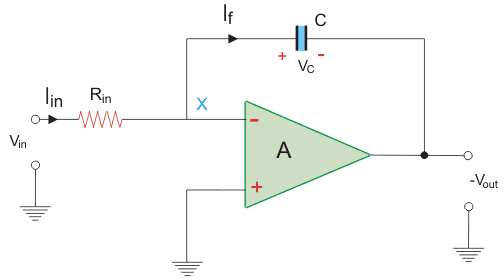## Op Amp Applications as Voltage to Current Converter

An op-amp with a negative feedback is generally used for voltage to current conversions. Below you can see the circuit diagram. We are not going into the details here, just we will discuss the circuit given below. The voltage is applied to the non-inverting terminal and the output is feedback to the inverting terminal. It is also grounded using a resistor.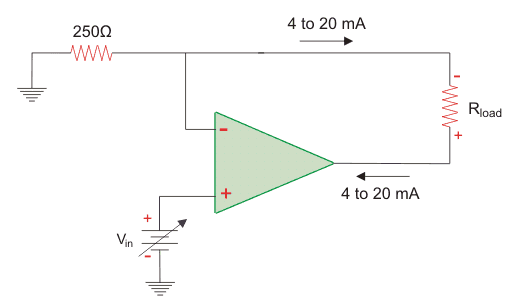## Op Amp Applications as Current to Voltage Converter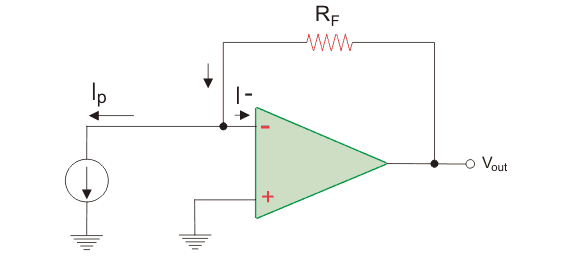Op-amp can be used as a current to voltage converter using a very simple circuit as shown above. All we need is a feedback resistance connected to the output of the op-amp. The current source is fed into the inverting terminal and the non-inverting terminal is grounded. Here the output voltage is proportional to the input current. As an ideal op-amp has infinite resistance, the current cannot flow through the op-amp. The current flows through the feedback resistance and the voltage across it depends on the current source.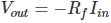## Op Amp Applications as Logarithmic Amplifier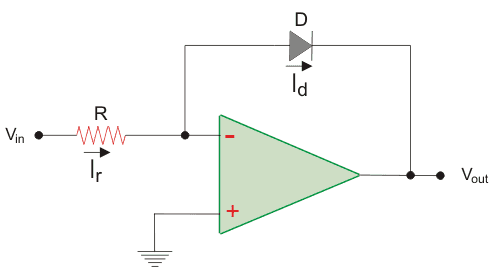The logarithmic amplifier using op-amp is made by using a diode instead of a resistance in the feedback loop. The non-inverting terminal is grounded and the input voltage is fed to the inverting terminal. The output voltage is proportional to the logarithm of the input voltage and hence can be used as a logarithmic amplifier.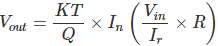## Op Amp Applications as Half Wave Rectifier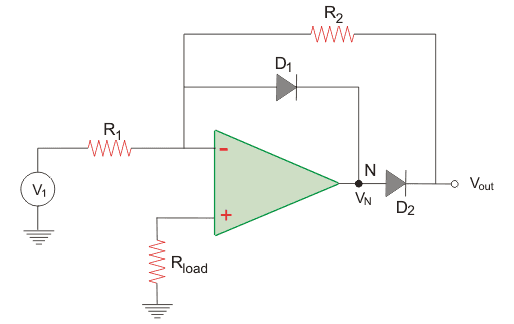The circuit diagram above shows the usage of an op-amp as a half wave rectifier. During the positive cycle of voltage, diode D2 is reversed biased as the positive signal is inverted by the op-amp. So there will be no output. However, during the negative cycle of the input voltage, the diode D2 is forward biased and conducts. Therefore the above circuit works as a half wave rectifier.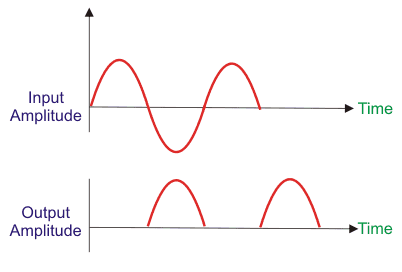## Op Amp Applications as Peak Detector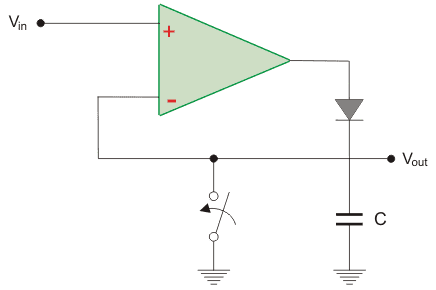The circuit above shows the use of op-amp as a peak detector. The circuit uses a diode and a capacitor. When Vout is more than Vin, the output is positive and the diode conducts. Whereas when Vout is less than Vin, the diode is reversed biased and does not conduct. The capacitor charges to the most positive value.

## Op Amp Applications as Voltage Comparator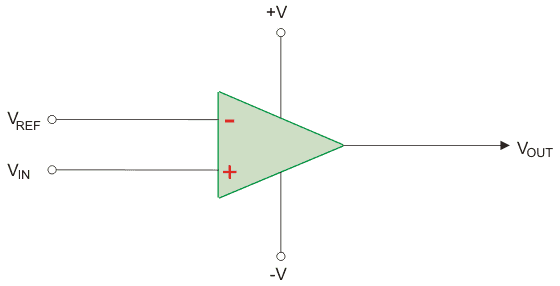This is perhaps the easiest to comprehend. Two voltage sources are applied to the two terminals of the op-amp. Let the reference voltage be applied to the inverting terminal and the voltage to be measured is applied to the non-inverting terminal. If the voltage applied is greater than the reference voltage, we will get a positive output, else we will get a negative output.

Want To Learn Faster? 🎓
Get electrical articles delivered to your inbox every week.
No credit card required—it’s 100% free.